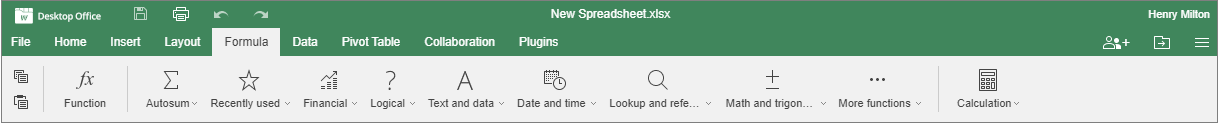## Formula tab

The Formula tab allows working easily with all functions.Figure. Formula tab.

Using this tab, you can:

• Insert functions using the Insert Function dialog window

• Quickly access Autosum formulas

• Access 10 recently used formulas

• Work with formulas classified into categories

• Work with named ranges

• Use the Calculation options: calculate the entire workbook, or the current worksheet only